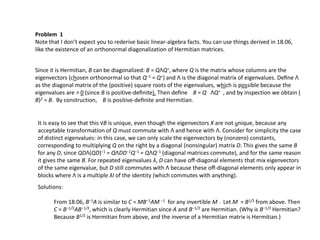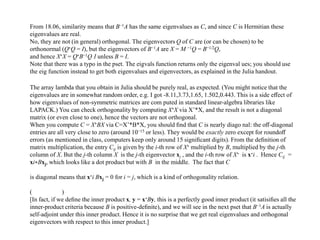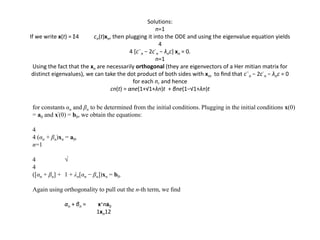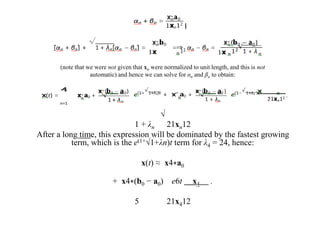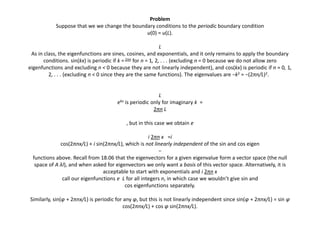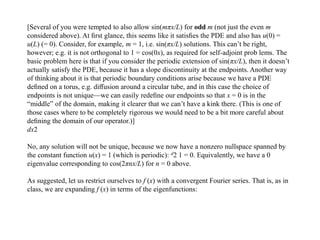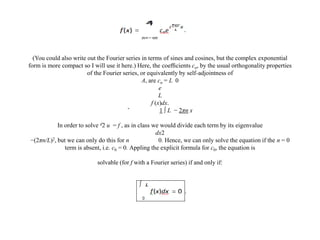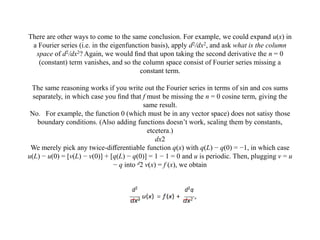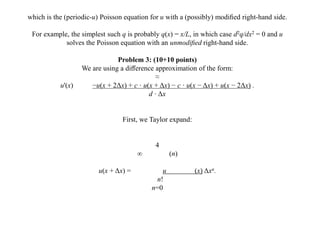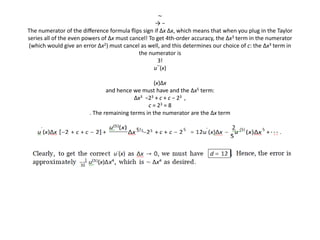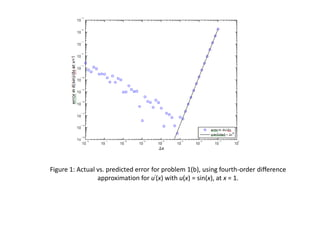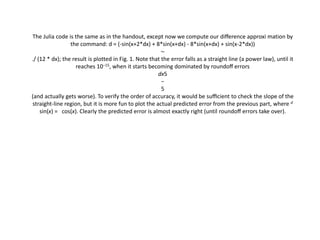1 of 14

### matrix theory and linear algebra.pptx

1. Matrix Theory and Linear Algebra
2. Since it is Hermitian, B can be diagonalized: B = QΛQ∗, where Q is the matrix whose columns are the eigenvectors (chosen orthonormal so that Q−1 = Q∗) and Λ is the diagonal matrix of eigenvalues. Deﬁne Λ as the diagonal matrix of the (positive) square roots of the eigenvalues, which is possible because the eigenvalues are > 0 (since B is positive-deﬁnite). Then deﬁne B = Q ΛQ∗ , and by inspection we obtain ( B)2 = B. By construction, B is positive-deﬁnite and Hermitian. Problem 1 Note that I don’t expect you to rederive basic linear-algebra facts. You can use things derived in 18.06, like the existence of an orthonormal diagonalization of Hermitian matrices. It is easy to see that this √B is unique, even though the eigenvectors X are not unique, because any acceptable transformation of Q must commute with Λ and hence with Λ. Consider for simplicity the case of distinct eigenvalues: in this case, we can only scale the eigenvectors by (nonzero) constants, corresponding to multiplying Q on the right by a diagonal (nonsingular) matrix D. This gives the same B for any D, since QDΛ(QD)−1 = QΛDD−1Q−1 = QΛQ−1 (diagonal matrices commute), and for the same reason it gives the same B. For repeated eigenvalues λ, D can have oﬀ-diagonal elements that mix eigenvectors of the same eigenvalue, but D still commutes with Λ because these oﬀ-diagonal elements only appear in blocks where Λ is a multiple λI of the identity (which commutes with anything). Solutions: From 18.06, B−1A is similar to C = MB−1AM −1 for any invertible M . Let M = B1/2 from above. Then C = B−1/2AB−1/2, which is clearly Hermitian since A and B−1/2 are Hermitian. (Why is B−1/2 Hermitian? Because B1/2 is Hermitian from above, and the inverse of a Hermitian matrix is Hermitian.)
3. From 18.06, similarity means that B−1A has the same eigenvalues as C, and since C is Hermitian these eigenvalues are real. No, they are not (in general) orthogonal. The eigenvectors Q of C are (or can be chosen) to be orthonormal (Q∗Q = I), but the eigenvectors of B−1A are X = M −1Q = B−1/2Q, and hence X∗X = Q∗B−1Q I unless B = I. Note that there was a typo in the pset. The eigvals function returns only the eigenval ues; you should use the eig function instead to get both eigenvalues and eigenvectors, as explained in the Julia handout. The array lambda that you obtain in Julia should be purely real, as expected. (You might notice that the eigenvalues are in somewhat random order, e.g. I got -8.11,3.73,1.65, 1.502,0.443. This is a side eﬀect of how eigenvalues of non-symmetric matrices are com puted in standard linear-algebra libraries like LAPACK.) You can check orthogonality by computing X∗X via X’*X, and the result is not a diagonal matrix (or even close to one), hence the vectors are not orthogonal. When you compute C = X∗BX via C=X’*B*X, you should ﬁnd that C is nearly diago nal: the oﬀ-diagonal entries are all very close to zero (around 10−15 or less). They would be exactly zero except for roundoﬀ errors (as mentioned in class, computers keep only around 15 signiﬁcant digits). From the deﬁnition of matrix multiplication, the entry Cij is given by the i-th row of X∗ multiplied by B, multiplied by the j-th column of X. But the j-th column X is the j-th eigenvector xj , and the i-th row of X∗ is x∗i . Hence Cij = xi∗Bxj, which looks like a dot product but with B in the middle. The fact that C is diagonal means that x∗i Bxj = 0 for i = j, which is a kind of orthogonality relation. ( ) [In fact, if we deﬁne the inner product x, y = x∗By, this is a perfectly good inner product (it satisiﬁes all the inner-product criteria because B is positive-deﬁnite), and we will see in the next pset that B−1A is actually self-adjoint under this inner product. Hence it is no surprise that we get real eigenvalues and orthogonal eigenvectors with respect to this inner product.]
4. Solutions: n=1 If we write x(t) = Σ4 cn(t)xn, then plugging it into the ODE and using the eigenvalue equation yields 4 4 [c¨n − 2c˙n − λnc] xn = 0. n=1 Using the fact that the xn are necessarily orthogonal (they are eigenvectors of a Her mitian matrix for distinct eigenvalues), we can take the dot product of both sides with xm to ﬁnd that c¨n − 2c˙n − λnc = 0 for each n, and hence cn(t) = αne(1+√1+λn)t + βne(1−√1+λn)t for constants αn and βn to be determined from the initial conditions. Plugging in the initial conditions x(0) = a0 and x'(0) = b0, we obtain the equations: 4 4 (αn + βn)xn = a0, n=1 4 √ 4 ([αn + βn] + 1 + λn[αn − βn])xn = b0. Again using orthogonality to pull out the n-th term, we ﬁnd αn + βn = x∗na0 1xn12
5. (note that we were not given that xn were normalized to unit length, and this is not automatic) and hence we can solve for αn and βn to obtain: √ 1 + λn 21xn12 After a long time, this expression will be dominated by the fastest growing term, which is the e(1+√1+λn)t term for λ4 = 24, hence: x(t) ≈ x4∗a0 + x4∗(b0 − a0) e6t x4 . 5 21x412
6. Problem Suppose that we we change the boundary conditions to the periodic boundary condition u(0) = u(L). L As in class, the eigenfunctions are sines, cosines, and exponentials, and it only remains to apply the boundary conditions. sin(kx) is periodic if k = 2πn for n = 1, 2, . . . (excluding n = 0 because we do not allow zero eigenfunctions and excluding n < 0 because they are not linearly independent), and cos(kx) is periodic if n = 0, 1, 2, . . . (excluding n < 0 since they are the same functions). The eigenvalues are −k2 = −(2πn/L)2. L ekx is periodic only for imaginary k = 2πn L , but in this case we obtain e i 2πn x =i cos(2πnx/L) + i sin(2πnx/L), which is not linearly independent of the sin and cos eigen − functions above. Recall from 18.06 that the eigenvectors for a given eigenvalue form a vector space (the null space of A λI), and when asked for eigenvectors we only want a basis of this vector space. Alternatively, it is acceptable to start with exponentials and i 2πn x call our eigenfunctions e L for all integers n, in which case we wouldn’t give sin and cos eigenfunctions separately. Similarly, sin(φ + 2πnx/L) is periodic for any φ, but this is not linearly independent since sin(φ + 2πnx/L) = sin φ cos(2πnx/L) + cos φ sin(2πnx/L).
7. [Several of you were tempted to also allow sin(mπx/L) for odd m (not just the even m considered above). At ﬁrst glance, this seems like it satisﬁes the PDE and also has u(0) = u(L) (= 0). Consider, for example, m = 1, i.e. sin(πx/L) solutions. This can’t be right, however; e.g. it is not orthogonal to 1 = cos(0x), as required for self-adjoint prob lems. The basic problem here is that if you consider the periodic extension of sin(πx/L), then it doesn’t actually satisfy the PDE, because it has a slope discontinuity at the endpoints. Another way of thinking about it is that periodic boundary conditions arise because we have a PDE deﬁned on a torus, e.g. diﬀusion around a circular tube, and in this case the choice of endpoints is not unique—we can easily redeﬁne our endpoints so that x = 0 is in the “middle” of the domain, making it clearer that we can’t have a kink there. (This is one of those cases where to be completely rigorous we would need to be a bit more careful about deﬁning the domain of our operator.)] dx2 No, any solution will not be unique, because we now have a nonzero nullspace spanned by the constant function u(x) = 1 (which is periodic): d2 1 = 0. Equivalently, we have a 0 eigenvalue corresponding to cos(2πnx/L) for n = 0 above. As suggested, let us restrict ourselves to f (x) with a convergent Fourier series. That is, as in class, we are expanding f (x) in terms of the eigenfunctions:
8. (You could also write out the Fourier series in terms of sines and cosines, but the complex exponential form is more compact so I will use it here.) Here, the coeﬃcients cn, by the usual orthogonality properties of the Fourier series, or equivalently by self-adjointness of A, are cn = L 0 e L f (x)dx. ˆ 1 ∫ L − 2πn x In order to solve d2 u = f , as in class we would divide each term by its eigenvalue dx2 −(2πn/L)2, but we can only do this for n 0. Hence, we can only solve the equation if the n = 0 term is absent, i.e. c0 = 0. Appling the explicit formula for c0, the equation is solvable (for f with a Fourier series) if and only if:
9. There are other ways to come to the same conclusion. For example, we could expand u(x) in a Fourier series (i.e. in the eigenfunction basis), apply d2/dx2, and ask what is the column space of d2/dx2? Again, we would ﬁnd that upon taking the second derivative the n = 0 (constant) term vanishes, and so the column space consist of Fourier series missing a constant term. The same reasoning works if you write out the Fourier series in terms of sin and cos sums separately, in which case you ﬁnd that f must be missing the n = 0 cosine term, giving the same result. No. For example, the function 0 (which must be in any vector space) does not satisy those boundary conditions. (Also adding functions doesn’t work, scaling them by constants, etcetera.) dx2 We merely pick any twice-diﬀerentiable function q(x) with q(L) − q(0) = −1, in which case u(L) − u(0) = [v(L) − v(0)] + [q(L) − q(0)] = 1 − 1 = 0 and u is periodic. Then, plugging v = u − q into d2 v(x) = f (x), we obtain
10. which is the (periodic-u) Poisson equation for u with a (possibly) modiﬁed right-hand side. For example, the simplest such q is probably q(x) = x/L, in which case d2q/dx2 = 0 and u solves the Poisson equation with an unmodiﬁed right-hand side. Problem 3: (10+10 points) We are using a diﬀerence approximation of the form: ≈ u'(x) −u(x + 2Δx) + c · u(x + Δx) − c · u(x − Δx) + u(x − 2Δx) . d · Δx First, we Taylor expand: 4 ∞ (n) u(x + Δx) = u (x) Δxn. n! n=0
11. ∼ → − The numerator of the diﬀerence formula ﬂips sign if Δx Δx, which means that when you plug in the Taylor series all of the even powers of Δx must cancel! To get 4th-order accuracy, the Δx3 term in the numerator (which would give an error Δx2) must cancel as well, and this determines our choice of c: the Δx3 term in the numerator is 3! u'''(x) (x)Δx and hence we must have and the Δx5 term: Δx3 −23 + c + c − 23 , c = 23 = 8 . The remaining terms in the numerator are the Δx term
12. Figure 1: Actual vs. predicted error for problem 1(b), using fourth-order diﬀerence approximation for u'(x) with u(x) = sin(x), at x = 1.
13. The Julia code is the same as in the handout, except now we compute our diﬀerence approxi mation by the command: d = (-sin(x+2*dx) + 8*sin(x+dx) - 8*sin(x+dx) + sin(x-2*dx)) ∼ ./ (12 * dx); the result is plotted in Fig. 1. Note that the error falls as a straight line (a power law), until it reaches 10−15, when it starts becoming dominated by roundoﬀ errors dx5 − 5 (and actually gets worse). To verify the order of accuracy, it would be suﬃcient to check the slope of the straight-line region, but it is more fun to plot the actual predicted error from the previous part, where d sin(x) = cos(x). Clearly the predicted error is almost exactly right (until roundoﬀ errors take over).
14. Thank You